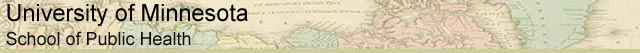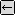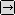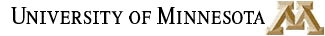### MATH REVIEW: USEFUL MATH FOR EVERYONE

SECTION 3.2. WHAT IS AN EXPONENT?back to Exponents, Page 1

First let's look at how to work with variables to a given power, such as a3.

There are five rules for working with exponents:

1. am * an = a(m+n)

2. (a * b)n = an * bn

3. (am)n = a(m * n)

4. am / an = a(m-n)

5. (a/b)n = an / bn

Let's look at each of these in detail.

1. am * an = a(m+n) says that when you take a number, a, multiplied by itself m times, and multiply that by the same number a multiplied by itself n times, it's the same as taking that number a and raising it to a power equal to the sum of m + n.

Here's an example where

a = 3
m = 4
n = 5

am * an = a(m+n)

34 * 35 = 3(4+5) = 39 = 19,683

2. (a * b)n = an * bn says that when you multiply two numbers, and then multiply that product by itself n times, it's the same as multiplying the first number by itself n times and multiplying that by the second number multiplied by itself n times.

Let's work out an example where

a = 3
b = 6
n = 5

(a * b)n = an * bn

(3 * 6)5 = 35 * 65

185 = 35 * 65 = 243 * 7,776 = 1,889,568

3. (am)n = a(m * n) says that when you take a number, a , and multiply it by itself m times, then multiply that product by itself n times, it's the same as multiplying the number a by itself m * n times.

Let's work out an example where

a = 3
m = 4
n = 5

(am)n = a(m * n)

(34)5 = 3(4 * 5) = 320 = 3,486,784,401

4. am / an = a(m-n) says that when you take a number, a, and multiply it by itself m times, then divide that product by a multiplied by itself n times, it's the same as a multiplied by itself m-n times.

Here's an example where

a = 3
m = 4
n = 5

am / an = a(m-n)

34 / 35 = 3(4-5) = 3-1 (Remember how to raise a number to a negative exponent.)

34 / 35 = 1 / 31 = 1/3

5. (a/b)n = an / bn says that when you divide a number, a by another number, b, and then multiply that quotient by itself n times, it is the same as multiplying the number by itself n times and then dividing that product by the number b multiplied by itself n times.

Let's work out an example where

a = 3
b = 6
n = 5

(a/b)n = an / bn

(3/6)5 = 35 / 65

Remember 3/6 can be reduced to 1/2. So we have:

(1/2)5 = 243 / 7,776 = 0.03125

Understanding exponents will prepare you to use logarithms.to Logarithms